#### Chapter 2 Exponents of Real Numbers R.D. Sharma Solutions for Class 9th Math Exercise 2.2

Exercise 2.2

1. Assuming that x, y, z are positive real numbers, simplify each of the following: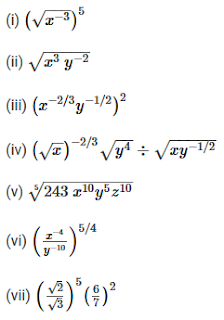Solution

We have to simplify the following, assuming that x,y,z are positive real numbers.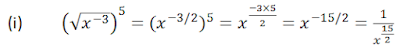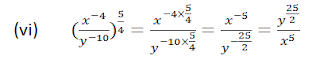2. Simplify

Solution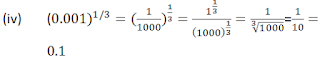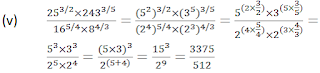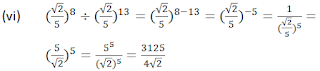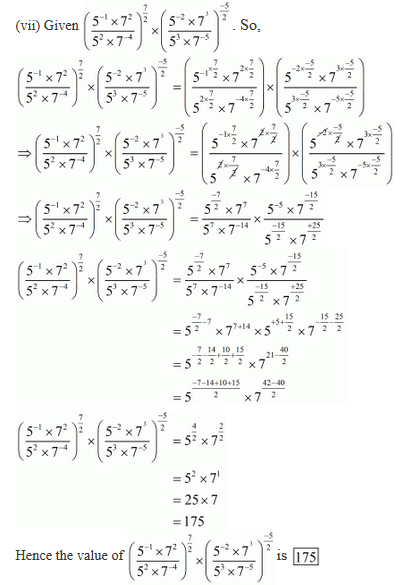3. Prove ThatSolution

(i)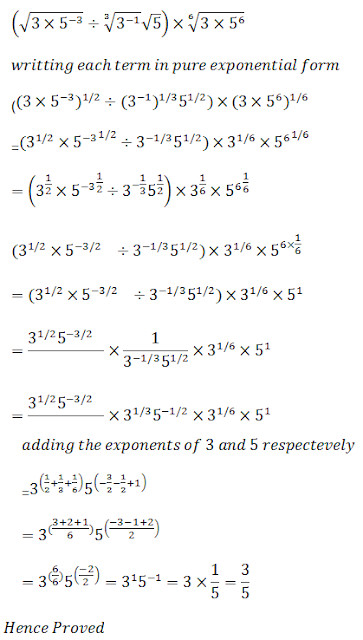(ii)(iii)(iv)(v)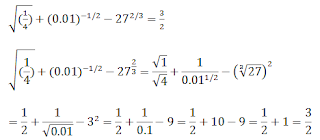(vi)(vii)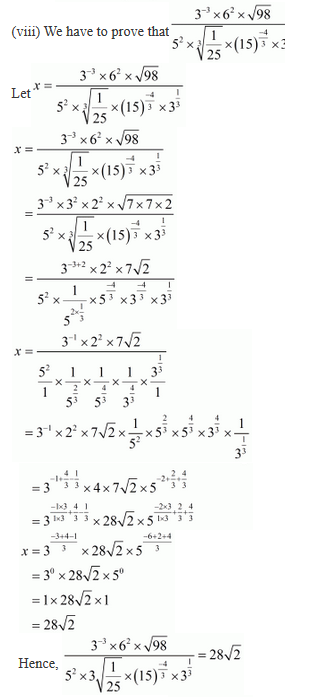(ix)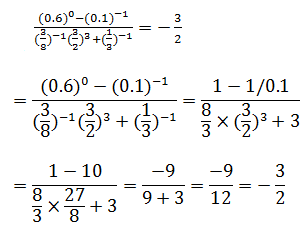4. Show that

Solution

(i)(ii)(iii)(iv)(v)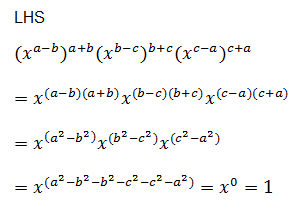(vi)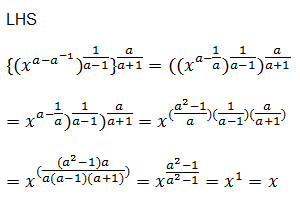(vii)(viii)Level - 2

5.Solution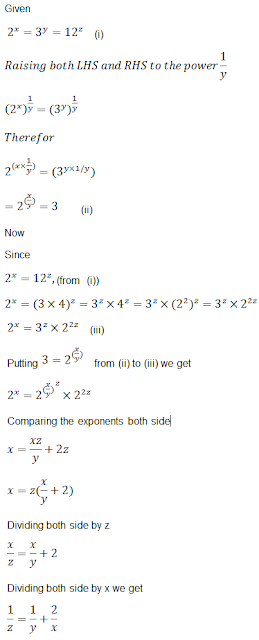6.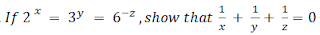Solution7.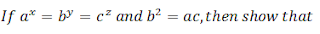Solution8.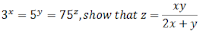Solution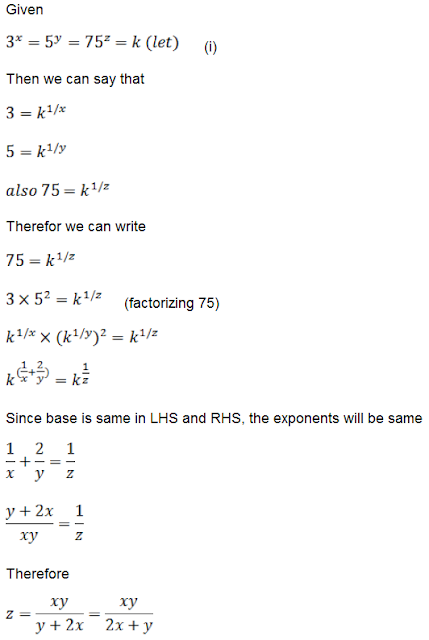9.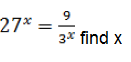Solution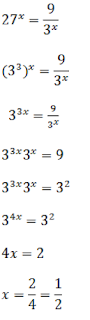10.  Find x in following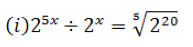(iii) (3/5)x(5/3)x = 125/27
(iv) 5x-2×32x-3 = 13
(v) 2x-7×5x-4 = 125/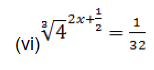(vii) 52x+3 = 1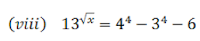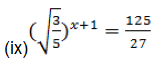Solution

(i)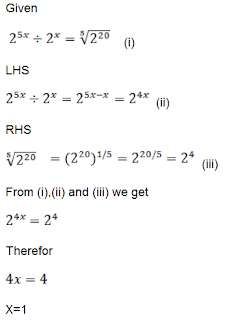(ii)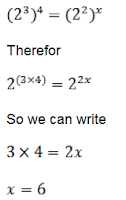(iii)(iv)(v)(vi)(vii)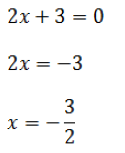(viii)(ix)11. If x = 21/3+32/3 show that x3 - 6x = 6

Solution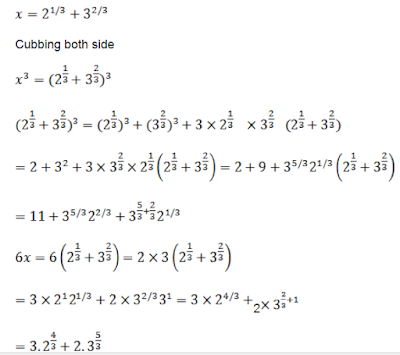12. Determine (8xx)x, If 9x+2 = 240 + 9x

Solution13. 3x+1 = 9x-2 Find the value of 21+x

Solution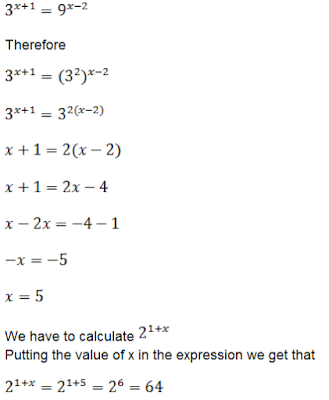14. If 34x = 81-1 and 101/y = 0.0001, find the value of 2-x+4y

Solution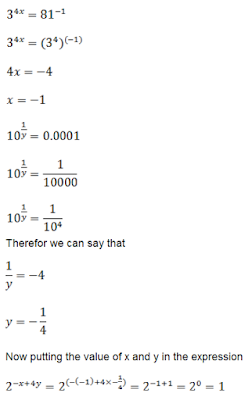15. If 53x = 125, and 10= 0.001, find x and y.

Solution16. Solve the following equations:
(i) 3x+1 = 27×34

Solution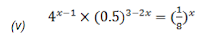Solution18. If a and b are different positive primes such thatSolution

(i)(ii)19. If 2x×3y×5= 2160, find x, y and z. Hence, compute the value of 3x×2-y×5-z

Solution20. If 1176 = 2a×3b×7find a,b,c and compute 2a×3b×7-c

Solution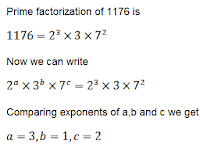21. Simplify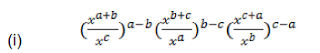Solution

(i)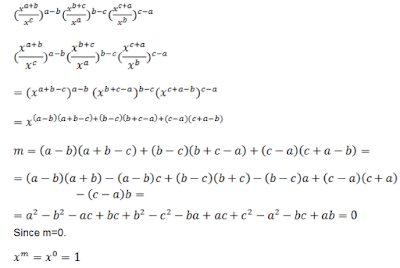(ii)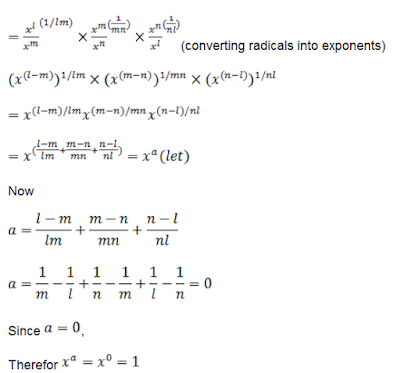22.  Show thatSolution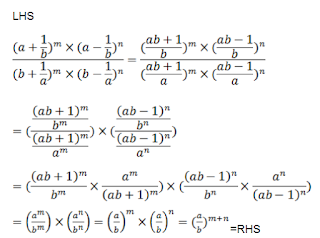23.
(i)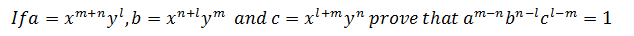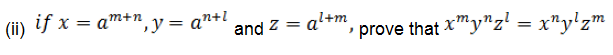Solution

(i)(ii)